### 如何处理矫正GEO数据批次效应（batch effect）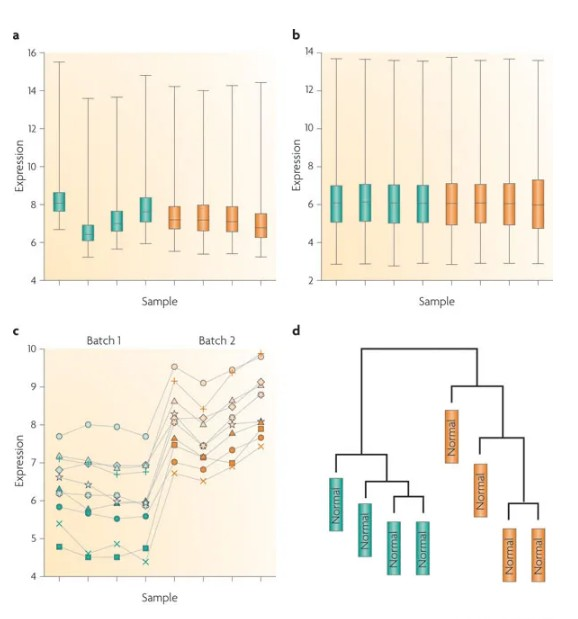### 矫正批次效应

#### 1.使用sva的中combat包来校正批次效应

`# 安装包,提前添加镜像，加快安装速度if (!requireNamespace("BiocManager", quietly=TRUE)){  install.packages("BiocManager")}options(BioC_mirror="https://mirrors.tuna.tsinghua.edu.cn/bioconductor")local({r <- getOption("repos")  r["CRAN"] <- "http://mirrors.tuna.tsinghua.edu.cn/CRAN/"   options(repos=r)}) BiocManager::install("sva")BiocManager::install("bladderbatch")library(sva)library(bladderbatch)library('Biobase')library('GEOquery')data(bladderdata)#bladder 的属性是EsetExpressionSet，所以可以用pData和exprs方法pheno <- pData(bladderEset) # 注释信息edata <- exprs(bladderEset) # 表达矩阵`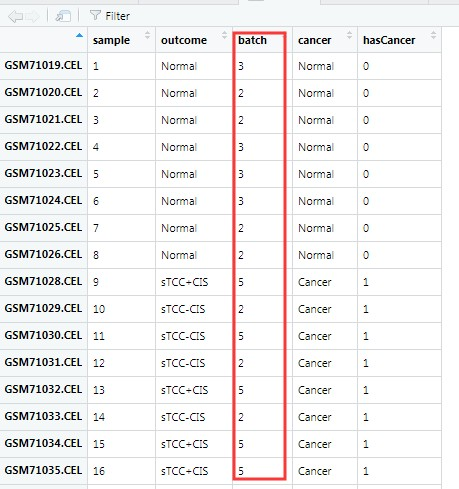`dist_mat <- dist(t(edata))clustering <- hclust(dist_mat, method = "complete")par(mfrow=c(2,1))plot(clustering, labels = pheno\$batch)plot(clustering, labels = pheno\$cancer)`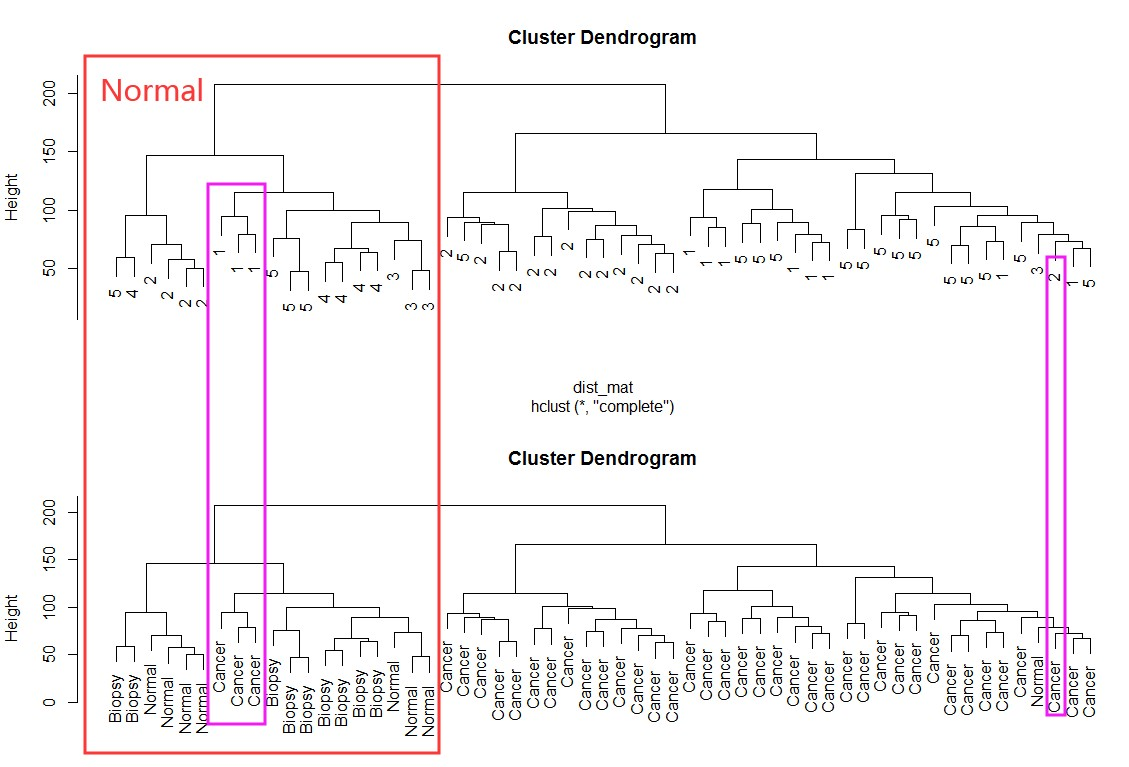校正批次效应：model可以有也可以没有，如果有，也就是告诉combat，有些分组本来就有差别，不要给我矫枉过正！

`#再做一个分组列，用于批次效应中排除项。pheno\$hasCancer <- as.numeric(pheno\$cancer == "Cancer")  #分组模型model <- model.matrix(~hasCancer, data = pheno)combat_edata <- ComBat(dat = edata, batch = pheno\$batch, mod = model)dist_mat_combat <- dist(t(combat_edata))clustering_combat <- hclust(dist_mat_combat, method = "complete")par(mfrow=c(2,1))plot(clustering_combat, labels = pheno\$batch)plot(clustering_combat, labels = pheno\$cancer)`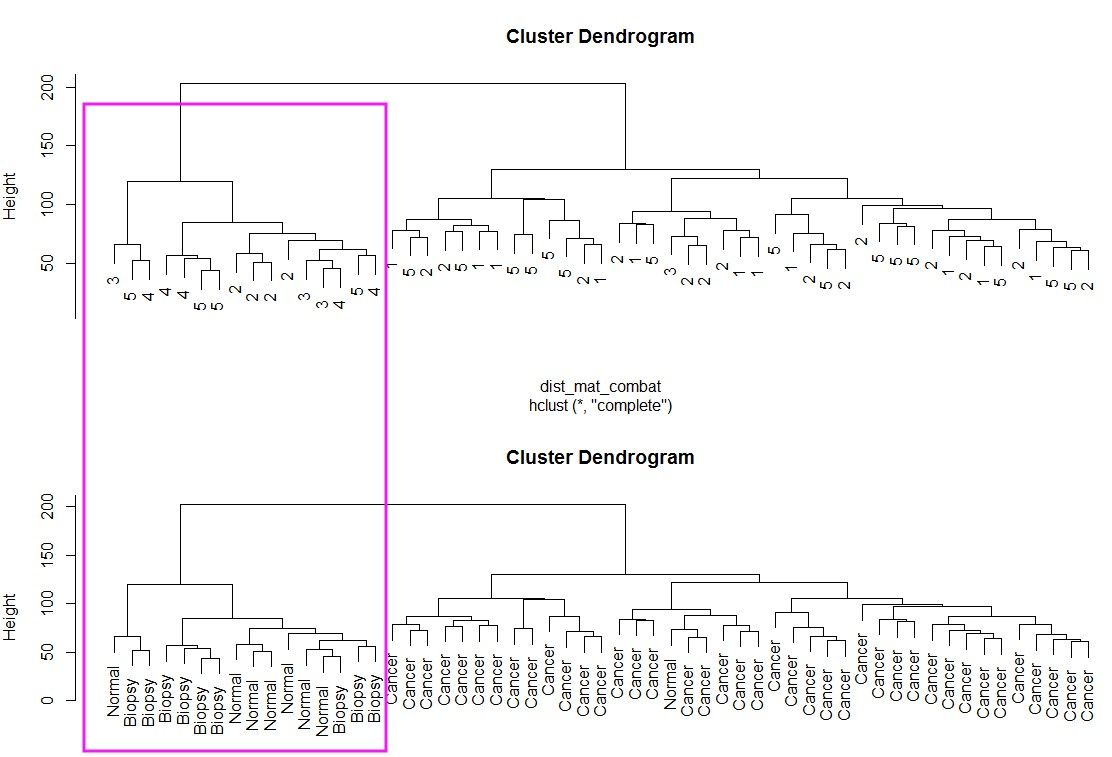#### 2.使用 limma 的 removeBatchEffect 函数

`dat = edata, batch = pheno\$batchlibrary("limma")design <- model.matrix(~pheno\$batch+pheno\$hasCancer)limma.edata <- removeBatchEffect(edata,                                batch = pheno\$batch,                                design = design)dist_mat_limma <- dist(t(limma.edata))clustering_limma <- hclust(dist_mat_limma, method = "complete")par(mfrow=c(2,1))plot(clustering_limma, labels = pheno\$batch)plot(clustering_limma, labels = pheno\$cancer)`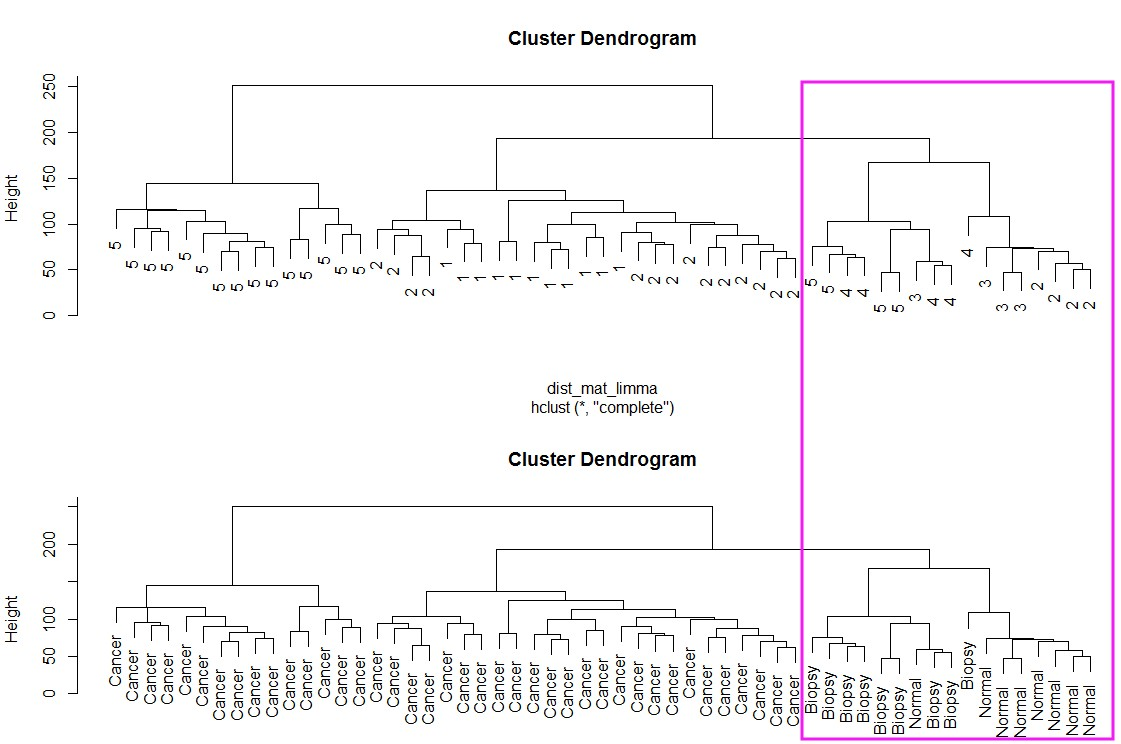## 更多生物信息课程：

1. 文章越来越难发？是你没发现新思路，基因家族分析发2-4分文章简单快速，学习链接：基因家族分析实操课程基因家族文献思路解读

2. 转录组数据理解不深入？图表看不懂？点击链接学习深入解读数据结果文件，学习链接：转录组（有参）结果解读转录组（无参）结果解读

3. 转录组数据深入挖掘技能-WGCNA，提升你的文章档次，学习链接：WGCNA-加权基因共表达网络分析

4. 转录组数据怎么挖掘？学习链接：转录组标准分析后的数据挖掘转录组文献解读

6. 生物信息入门到精通必修基础课：linux系统使用biolinux搭建生物信息分析环境linux命令处理生物大数据perl入门到精通perl语言高级R语言画图R语言快速入门与提高python语言入门到精通

7. 医学相关数据挖掘课程，不用做实验也能发文章：TCGA-差异基因分析GEO芯片数据挖掘 GEO芯片数据不同平台标准化 、GSEA富集分析课程TCGA临床数据生存分析TCGA-转录因子分析TCGA-ceRNA调控网络分析

#### 9.全部课程可点击：组学大讲堂视频课程

• 发表于 2019-11-19 15:45
• 阅读 ( 20030 )
• 分类：GEO

## 作家榜 »

1.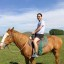omicsgene 603 文章
2.安生水 280 文章
3.Daitoue 167 文章
4.生物女学霸 120 文章
5.红橙子 78 文章
6.CORNERSTONE 72 文章
7.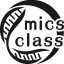生信老顽童 53 文章
8.landy 37 文章